# An aqueous phase reversible reaction ? ⇌ ? + ? is carried out in a batch...

An aqueous phase reversible reaction ? ⇌ ? + ? is carried out in a batch reactor. The forward reaction is first order wrt A and the backward reaction is first order wrt each component. Initial concentration of A is 1 mol/litre and no R and S are present initially. Derive an expression for ?? ⁄??. What is the maximum allowable conversion in the reactor? If this reaction happens in gas phase (constant pressure), derive an expression for ?? ⁄??. Assume same rate law and initial concentrations.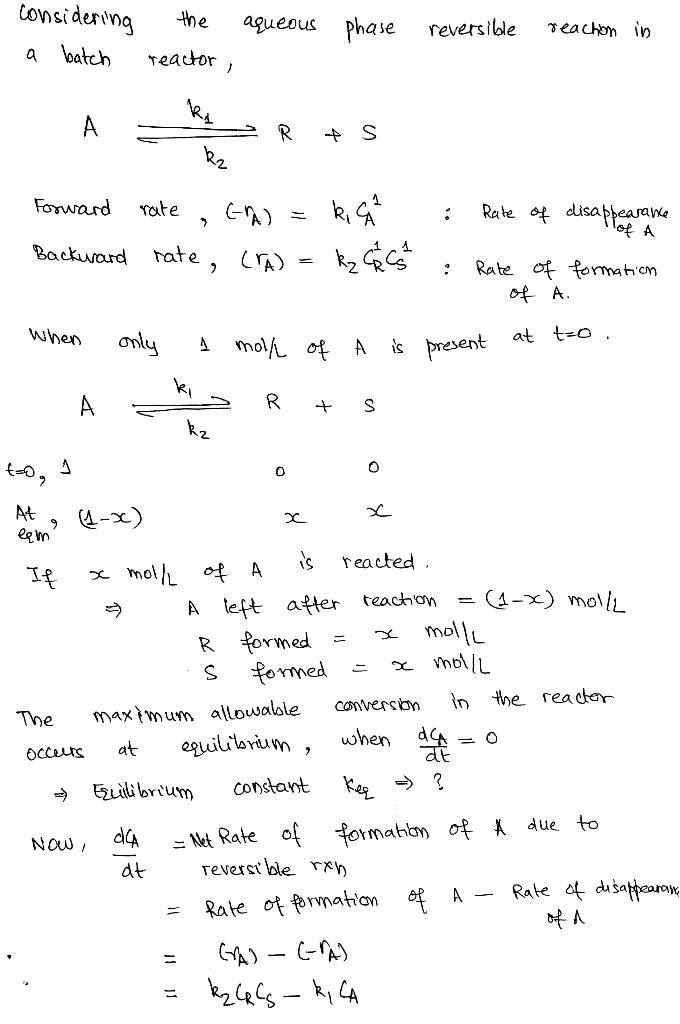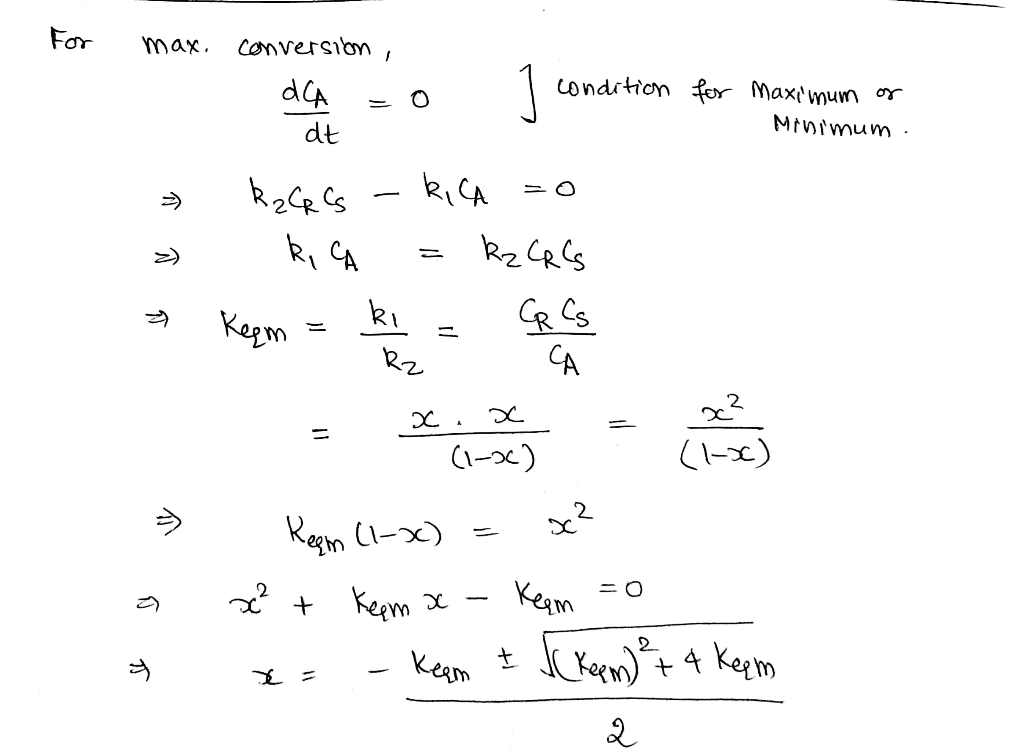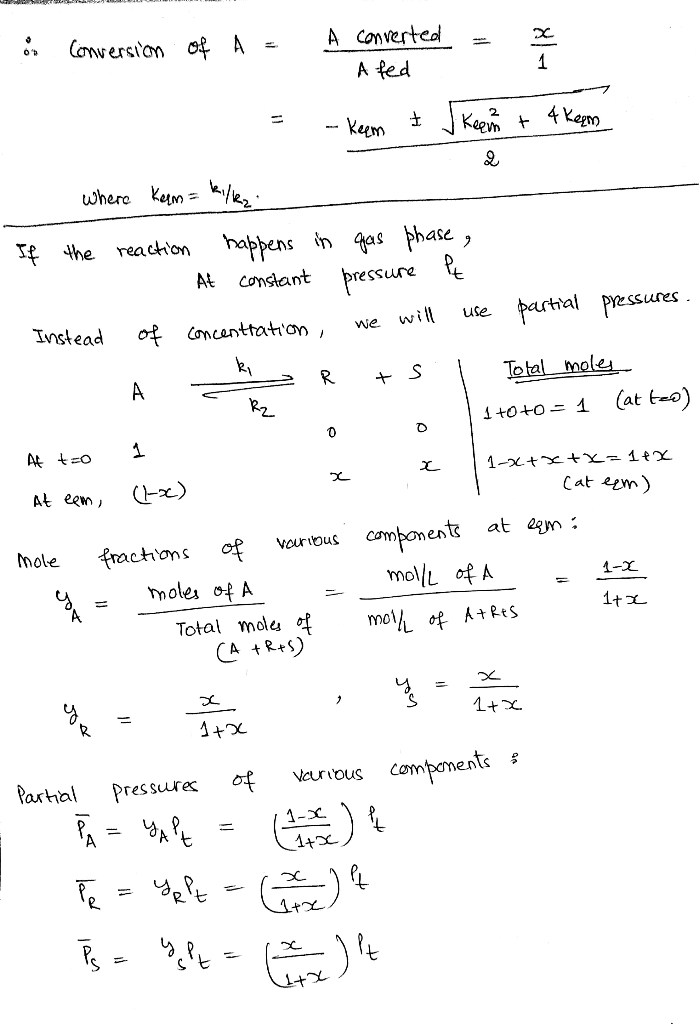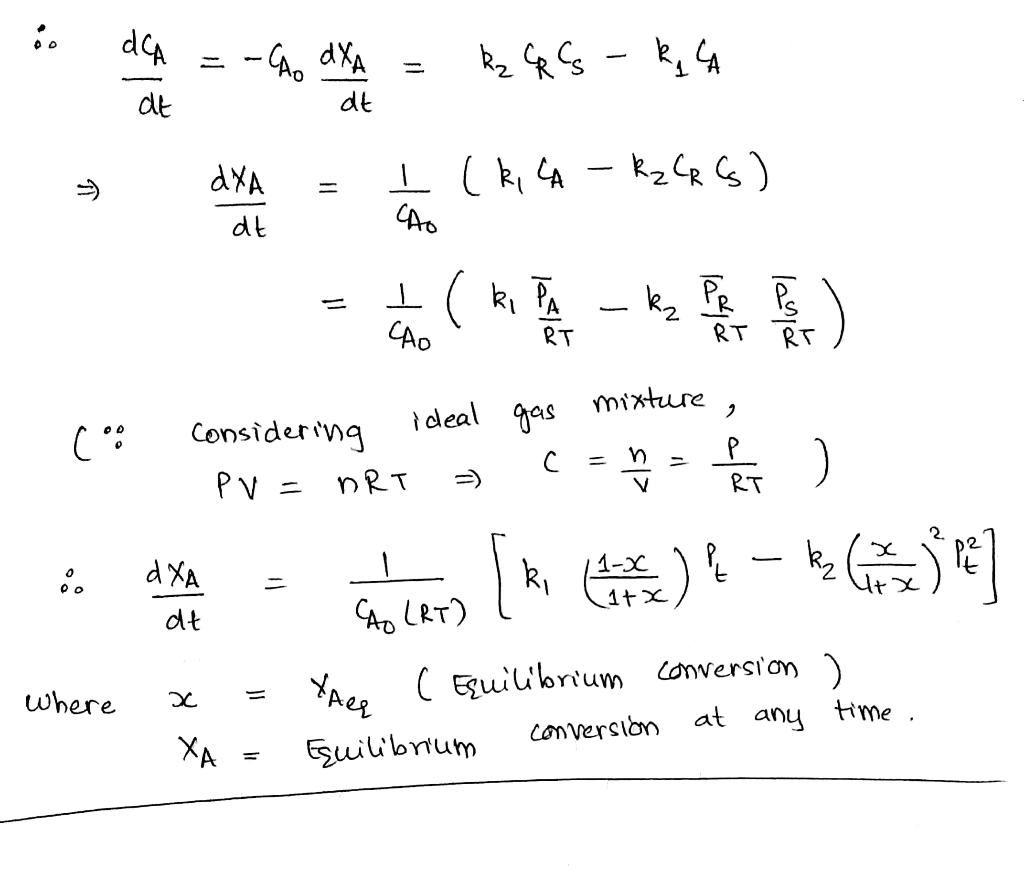##### Add Answer to: An aqueous phase reversible reaction ? ⇌ ? + ? is carried out in a batch...
Similar Homework Help Questions
• ### The second order gas phase irreversible reaction: 2.4-B is carried out in an isothermal batch reactor...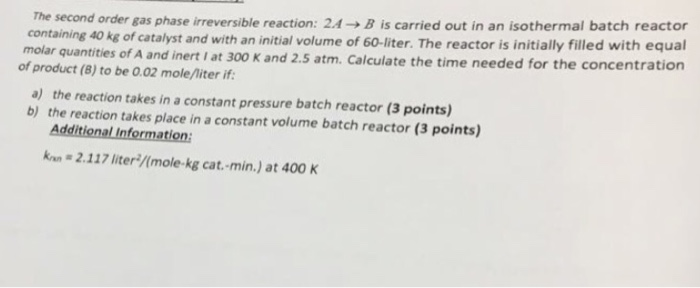The second order gas phase irreversible reaction: 2.4-B is carried out in an isothermal batch reactor containing 40 kg of catalyst and with an initial volume of 60-liter. The reactor is initially filled with equal molar quantities of A and inert I at 300 K and 2.5 atm. Calculate the time needed for the concentration of product (B) to be 0.02 mole/liter if: a) the reaction takes in a constant pressure batch reactor (3 points) b) the reaction takes place...

• ### The production of methyl bromide is an irreversible liquid-phase reaction that follows an elementary rate law. The reaction CNBr (A) + CH3NH2 (B)àCH3Br (C) + NCNH2 (D) is carried out isothermally in...

The production of methyl bromide is an irreversible liquid-phase reaction that follows an elementary rate law. The reaction CNBr (A) + CH3NH2 (B)àCH3Br (C) + NCNH2 (D) is carried out isothermally in a semi-batch reactor. A solution of methylamine (B) in extra dry ethanol at a concentration of 0.025 mol/L is to be fed at a rate of 0.05 L/s to an solution of bromine cyanide (A) in extra dry ethanol contained in a glass-lined reactor. The initial volume of...

• ### 4a)For the gas-phase reaction C H, isothermally in a CiH, → Collio or konstant-volume batch reactorl what sho...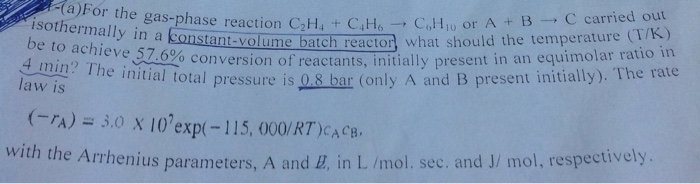4a)For the gas-phase reaction C H, isothermally in a CiH, → Collio or konstant-volume batch reactorl what should the temperature ( ,meve. .6% conversion of reactants, initially present in law is enitial total pressure is Q& bar (only A and B present initially). The rate -A) 3.0 X 10'exp(-115, 000/RT)CAcB. an equimolar ratio in with the e Arrhenius parameters, A and E, in L /mol. sec. and J/ mol, respectively (D) USing the same temperature as i conversion of ethane,...

• ### The elementary, reversible gas phase reaction A B is to be carried out in a CSTR with heat exchange. Pure A is fed to t...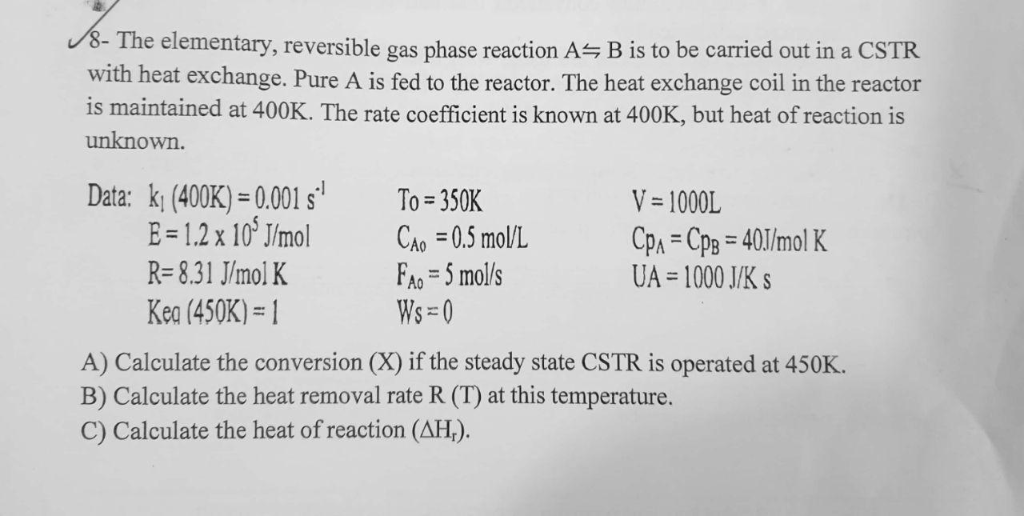The elementary, reversible gas phase reaction A B is to be carried out in a CSTR with heat exchange. Pure A is fed to the reactor. The heat exchange coil in the reactor is maintained at 400K. The rate coefficient is known at 400K, but heat of reaction is unknown. Data: k1(400K) = 0.001 s.! V-1000L To=350K R-831 J/mol K h 5 mol's UA 1000 J/K Kea (450K-1 A) Calculate the conversion (X) if the steady state CSTR is operated...

• ### 3. The endothemic irreversible 2nd order reaction A +B C is carried out in a pressurised...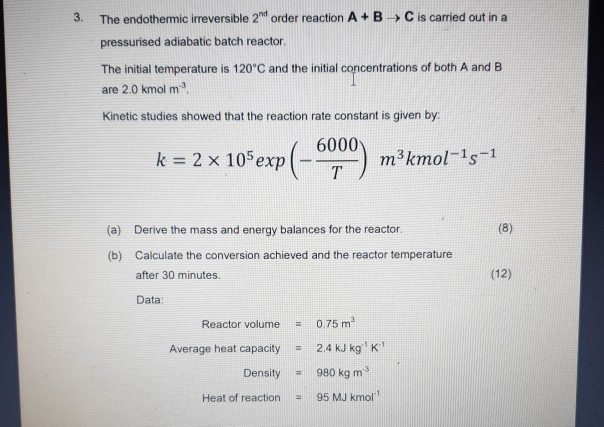3. The endothemic irreversible 2nd order reaction A +B C is carried out in a pressurised adiabatic batch reactor. The initial temperature is 120°C and the initial concentrations of both A and B are 2.0 kmol m2 Kinetic studies showed that the reaction rate constant is given by: 6000 k 2 x 105 exp(- m3kmol 1s1 T (8) (a) Derive the mass and energy balances for the reactor. Calculate the conversion achieved and the reactor temperature (b) (12) after 30...

• ### . Question 2: The elementary, reversible, organic, liquid-phase reaction is carried out adiabatically in a CSTR...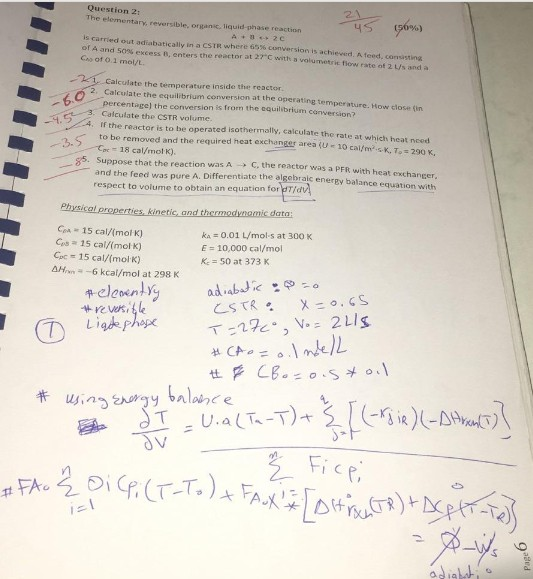. Question 2: The elementary, reversible, organic, liquid-phase reaction is carried out adiabatically in a CSTR where 65% conversion is achiewd Ated'anonn of A and 50% excess 8, enters the reactor at 27°C with a volumetric flow rate of 2 L/s and a Cos of O.1 mol/L culate the temperature inside the reactor 2. Calculate the equilibrium conversion at the operating temperature. How close (in 3. Calculate the CSTR volume percentage) the conversion is from the equilsbrium conversion? If the...

• ### Question 1: Design of isothermal reactors 30 Marks The irreversible, gas-phase reaction A+B D is to be carried out...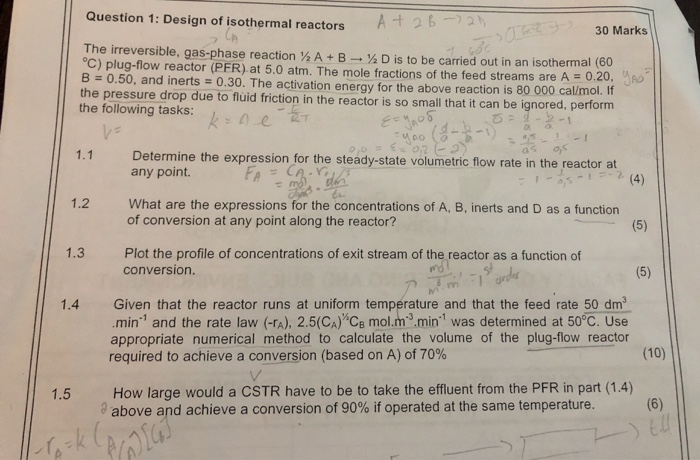Question 1: Design of isothermal reactors 30 Marks The irreversible, gas-phase reaction A+B D is to be carried out in an isotherma °C) plug-flow reactor (PFR) at 5.0 atm. The mole fractions of the feed streams are A 0 B 0.50, and inerts 0.30. The activation energy for the above reaction is 80 000 cal/mol. the pressure drop due to fluid friction in the reactor is so small that it can be ignored, perform the following tasks: 2T a s...

• ### An acid-catalyzed irreversible liquid-phase reaction A B is carried out adiabatically in a CSTR. The reaction is second...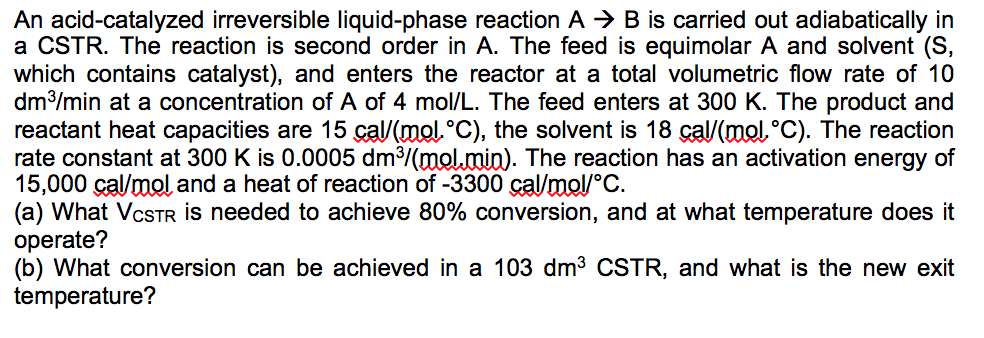An acid-catalyzed irreversible liquid-phase reaction A B is carried out adiabatically in a CSTR. The reaction is second order in A. The feed is equimolar A and solvent (S, which contains catalyst), and enters the reactor at a total volumetric flow rate of 10 dm3/min at a concentration of A of 4 mol/L. The feed enters at 300 K. The product and reactant heat capacities are 15 cal/(mol°C), the solvent is 18 cal/(mol°C). The reaction rate constant at 300 K...

• ### energy il Camor P5-30 Pressure Drop. The gas phase reaction A+B C+D is carried out isothermally...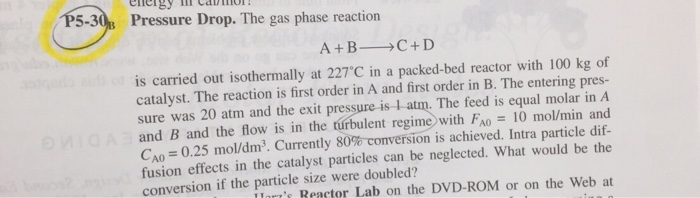energy il Camor P5-30 Pressure Drop. The gas phase reaction A+B C+D is carried out isothermally at 227 C in a packed-bed reactor with 100 kg of catalyst. The reaction is first order in A and first order in B. The entering pres- sure was 20 atm and the exit pressure is 1 atm. The feed is equal molar in A onigA and B and the flow is in the turbulent regime with FA0 10 mol/min and CAO= 0.25 mol/dm3....

• ### QUESTION 5: 10 marks An elementary aqueous phase reaction A --> B+C is to be carried...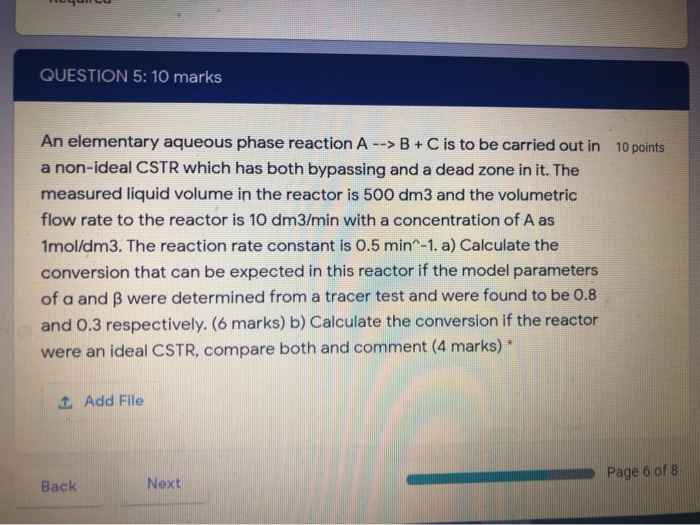QUESTION 5: 10 marks An elementary aqueous phase reaction A --> B+C is to be carried out in 10 points a non-ideal CSTR which has both bypassing and a dead zone in it. The measured liquid volume in the reactor is 500 dm3 and the volumetric flow rate to the reactor is 10 dm3/min with a concentration of A as Imol/dm3. The reaction rate constant is 0.5 min^-1. a) Calculate the conversion that can be expected in this reactor if...

Free Homework App